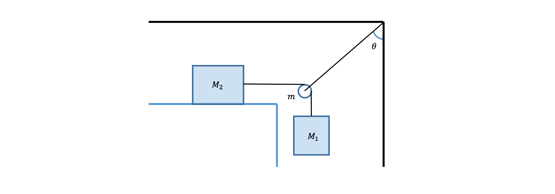# Problem: Consider the figure given below. Mass M 1 = 15 kg is free-hanging, supported by the tension in the rope. The mass M2 = 5 kg is at rest on the surface, under the influence of static friction with a coefficient μs = 0.4, which is balancing the tension in the rope pulling it to the right. In order for the rope to connect the masses, it passes over a pulley with mass m = 100 g supported by a rope anchored to the ceiling. In order for this system to be in equilibrium, what must the tension in the rope supporting the pulley be? Assume that the rope holding the pulley is attached to the center of the pulley, allowing it to freely change its angle θ, and that both ropes are massless.

🤓 Based on our data, we think this question is relevant for Professor Yust's class at Thomas Jefferson University.

###### Problem Details

Consider the figure given below. Mass M 1 = 15 kg is free-hanging, supported by the tension in the rope. The mass M2 = 5 kg is at rest on the surface, under the influence of static friction with a coefficient μs = 0.4, which is balancing the tension in the rope pulling it to the right. In order for the rope to connect the masses, it passes over a pulley with mass m = 100 g supported by a rope anchored to the ceiling. In order for this system to be in equilibrium, what must the tension in the rope supporting the pulley be? Assume that the rope holding the pulley is attached to the center of the pulley, allowing it to freely change its angle θ, and that both ropes are massless.